## Article: Inter-Rater Reliability – Calculating Kappa

1/12/2017 Reliability is the “consistency” or “repeatability” of your measures (William M.K. Trochim, Reliability) and, from a methodological perspective, is central to demonstrating that you’ve employed a rigorous approach to your project. There are a number of approaches to assess inter-rater reliability—see the Dedoose user guide for strategies to help your team build and maintain high levels of consistency—but today we would like to focus on just one, Cohen’s Kappa coefficient. So, brace yourself and let’s look behind the scenes to find how Dedoose calculates Kappa in the Training Center and find out how you can manually calculate your own reliability statistics if desired.

Kappa, k, is defined as a measure to evaluate inter-rater agreement as compared to the rate of agreement that can be expected by chance based on the overall coding decisions of each coder.

Basically, this just means that Kappa measures our actual agreement in coding while keeping in mind that some amount of agreement would occur purely by chance.

We can calculate Kappa with the following formula: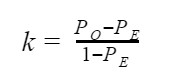HUH? The formula appears simple enough, but we’ll need some terms defined. What is PO? What is PE? To know that we first must have our counts of agreement and disagreement in coding, with these we can create the following table: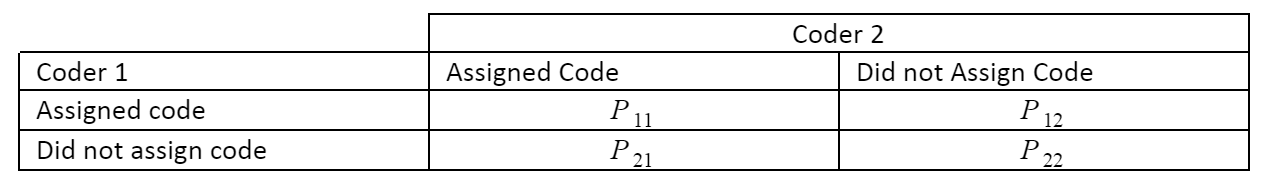Note that all P’s sum to 1 as they represent the relative frequencies of each case, thus they are divided by the total number of excerpts or sections of text that were coded.

Where PO is the percentage of agreement we actually observed (‘o’ for ‘observed’):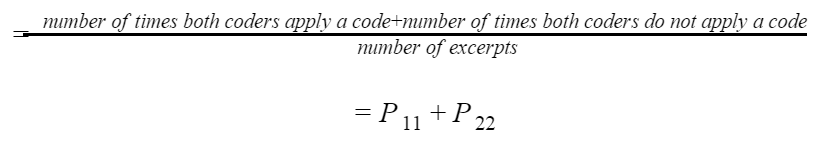And where PE is the percentage of agreement we would expect by chance (‘e’ for ‘expected’):

=number of times coder 2 applied the code*number of times coder 1 applied the code number of times coder 2 did not apply the code * the number of times coder 1 did not apply the code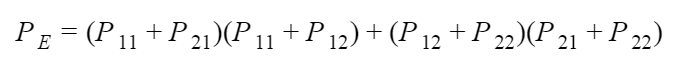Now we can use the formula: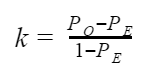Let’s do an example! Say we want to find our kappa statistic for a set of 50 excerpts that two coders coded with a single code and we have drawn up our table below with the counts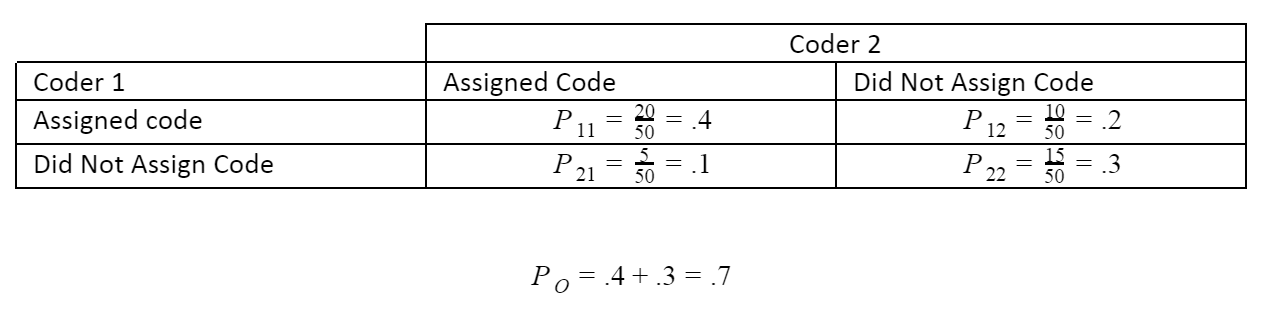Makes sense, right? They both coded the same 20 excerpts with the code and they both did not apply the code to 15 other excerpts, as such they agreed on when to apply the code (or not apply the code) on 70% of the excerpts.

Now, given the coding decisions by the two coders overall, what level of agreement would we expect to see by chance?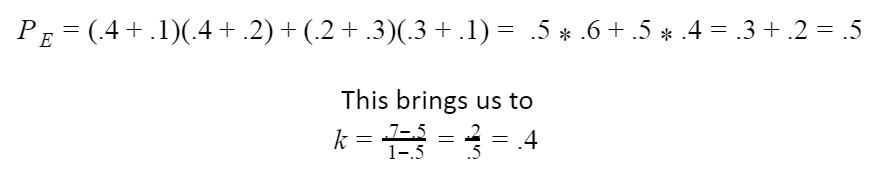See? It’s not so bad to calculate! In this case our k=.4….which actually isn’t so hot. Before we move forward, some things to note in passing: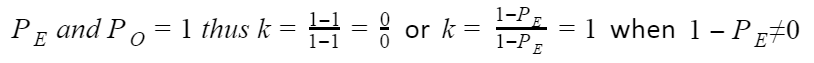• Both coders must code the same set of excerpts
• If the coders agreed on all excerpts, then k=undefined or 1 since
• If k is negative, then there is less agreement in coding than expected from chance.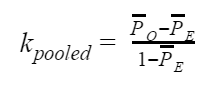Now that we know how to calculate a single kappa, we jump into the deep end and calculate pooled kappa for a set of codes, kpooled. De Vries et al. (2008) proposed using a pooled Kappa, kpooled, as an alternative to simply averaging Kappa values across your set of codes (and this is the approach we’ve taken in Dedoose).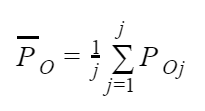Now this looks familiar, but just like before there are some symbols we haven’t defined yet: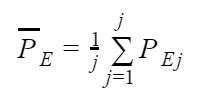We admit, that can look a bit intimidating if you’ve not spent time dealing with all the Greek letters involved in statistical notation. Yet, don’t fret, this just means that we are averaging all observed agreements for our codes in PO and all expected agreement by chance for our codes in PE. From there we just plug those averaged values into our familiar k formula and we’ll have our kpooled. So… let’s go through an example!

For conciseness, we are going to do a set of 3 codes and we’ll do the calculation for our PO and PE. First our original code: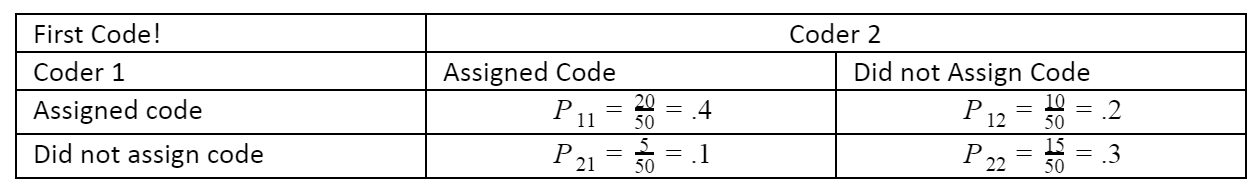From before, we know our PO=.7 and PE=.5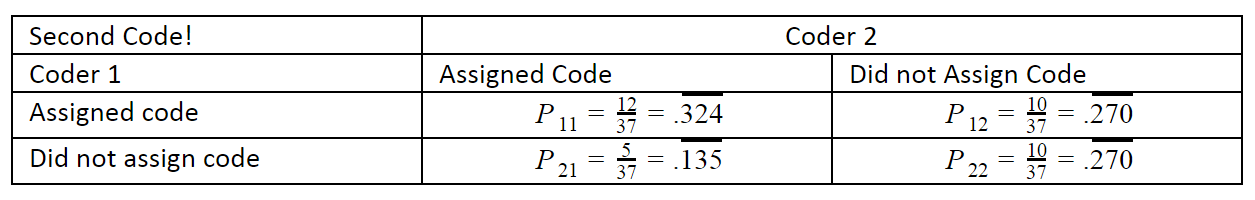Just for clarity, we’ll do the individual kappa statistic for this case: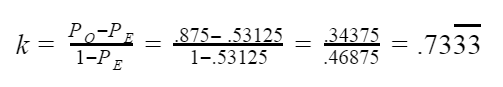Now, we’ve calculated all our PO and PE values, so let’s calculate our pooled kappa: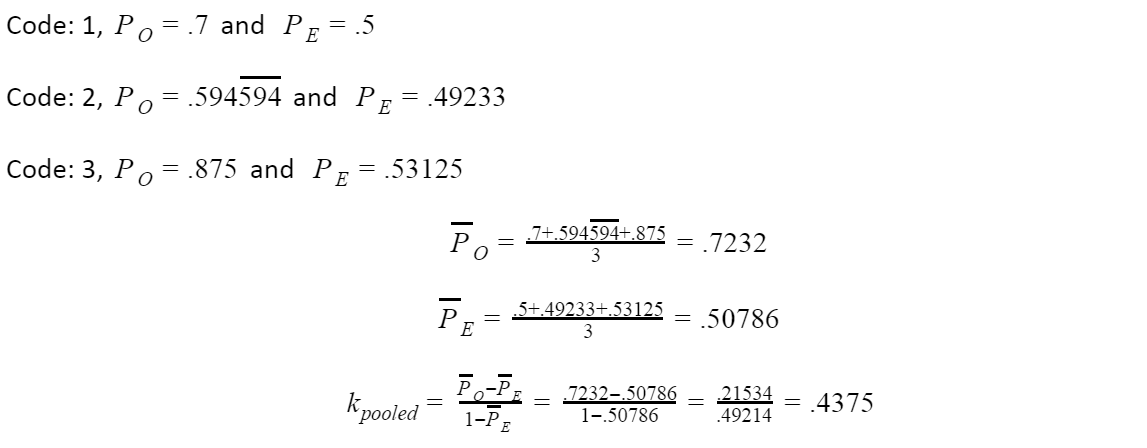Done! Conceptually it isn’t too bad, right? Manually calculating larger sets of codes and excerpts however can certainly be tedious if done by hand, but there are many tools to assist. Hope this helps and do send word if you want to see more articles like this? Got any questions? Let us know!

References

De Vries, H., Elliott, M. N., Kanouse, D. E., Teleki, S. S. (2008). Using Pooled Kappa to Summarize Interrater Agreement across Many Items. Field Methods, 20(3), 272-282.

Trochim, W. M. (2006). Reliability. Retrieved December 21, 2016, from http://www.socialresearchmethods.net/kb/reliable.php